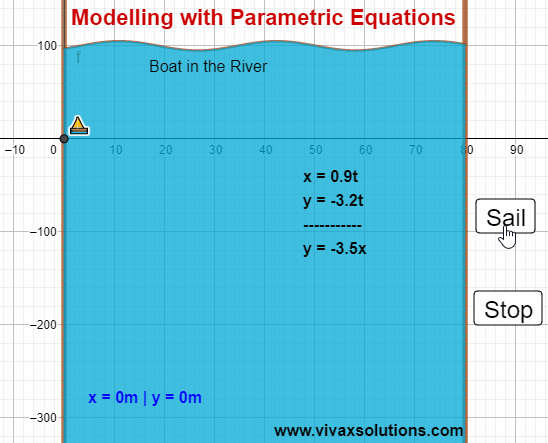### Modelling with Parametric Equations: A Level Pure MathematicsModelling with Parametric Equations for A Level pure mathematics

Parametric equations can be used to model real life situations. For example, the above animation shows how the path of a boat in river can be modelled by parametric equations.

The boat sails from west to east and the river flows from north to south in this case.

x and y are distances measured from the west and north respectively.

If the parameter is time, t,
x = 0.9t | y = -3.2t

The cartesian equation of the path is derived as follows:
x = 0.9t => t = x/0.9
y = -3.2(x/0.9)
y = -3.5x
Therefore, the path of the boat is a straight line.

You can practise the above interactively here: